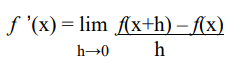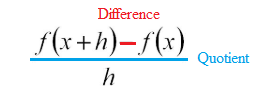# Difference Quotient: Definition & Example

## What is a Difference Quotient?

The difference quotient is one way to find a derivative or slope of a function. The formula is:It might look intimidating, but it’s easier to solve than it looks, because many of the terms cancel out.

It’s called a “difference quotient” because the formula has two parts: a difference (subtraction) in the numerator and a quotient (division) between the two parts:Watch the video for an overview and example:

## Example

Find the difference quotient for the following function: f(x) = 3x + 2

Step 1: Insert your function into the first part of the formula. In this step, I’m replacing the “f(x+h)” in the left hand part of the numerator with the actual given function, 3x + 2:Essentially, I just changed the “x” part of 3x + 2 with “x + h”.

Step 2: Replace the “f(x)” in the formula’s numerator. This part is easy, because you’re just copying the formula exactly as written in the question and pasting it in:Step 3: Use algebra to simplify. In most cases, things will start to cancel out, making the formula a little less ugly.

For this particular formula, the first step of simplifying is to remove the parentheses, to get:From here, you should be able to see that the following terms cancel out:

• 3x – 3x = 0
• 2 – 2 = 0

Leaving 3h / h = 3.

The difference quotient (aka the derivative or slope) is 3.

## Origins of the Difference Quotient

The formula is derived from the “rise/run” slope formula you’re probably familiar with from algebra:It’s the same formula, with a few substitutions. For example, instead of “y2” in the slope formula, you have “f(x + h)” in the difference quotient formula.

The full list of substitutions:

• f(x + h) → y2
• f(x) → y1
• x → x1
• x + h → x2
• h → change in x
• f(x + h) – f(x) → (y2 – y1)

Substituting those in to the slope formula, you get:## References

Difference Quotient. Retrieved October 13, 2019 from: https://www.csusm.edu/mathlab/documents/differencequotient-r6.pdf

CITE THIS AS:
Stephanie Glen. "Difference Quotient: Definition & Example" From StatisticsHowTo.com: Elementary Statistics for the rest of us! https://www.statisticshowto.com/derivatives/difference-quotient/